# Finite differences and second quantization

Most recent TBTK release at the time of writing: v1.0.3

Single-particle quantum mechanics is usually formulated using a differential equation such as the Schrödinger equation. The simplest way to solve this numerically is through the use of finite differences. While TBTK is designed for discrete problems formulated on second quantized form, this includes the possibility to work with finite difference schemes. In this post, we, therefore, go through the basic principles of finite differences and how to arrive at a discretized version of the Schrödinger equation. We then describe how to translate this to a second quantized form.

While we focus on the Schrödinger equation, the methods discussed here can be applied to any finite difference scheme and should therefore be of broad interest also beyond quantum mechanics. In particular, the ease with which models are extended beyond one dimension should be of interest for anyone implementing finite difference calculations in general.

## Finite differences

### First derivative

Finite differences are simple to understand if we remind ourselves of the definition of the derivative of a function,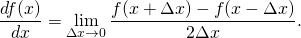As the name implies, finite differences result when the derivative is instead approximated by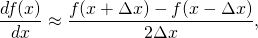for some finite discretization length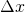.

The finite difference scheme naturally defines a uniform grid containing the points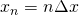for integer. Moreover, since the finite difference approximation should be understood to be valid for each of these points, it really is a set of linear equations of the form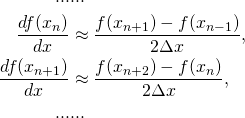Using matrix notation, this can also be expressed as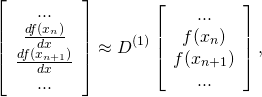where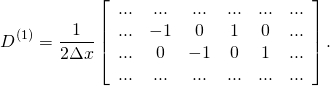Here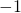andappear right below and above the diagonal, respectively.

For the problem to be possible to put into a computer, the number of discretization points need to be finite, for examplefor some finite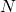. The matrix therefore necessarily have to be truncated, which means that the first and last row in fact corresponds to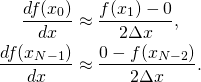That is, by simply truncating the matrix we imply the boundary conditions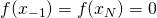. Other boundary conditions can be added back into the formulation, but for simplicity we will consider these boundary conditions here.

### Forward, centered, and backward differences

To be more specific, the finite difference scheme above is called a centered difference since it is formulated symmetrically around the point at which the derivative is approximated. For functions with well defined derivative we could equivalently have formulated the derivative using any of the three expressions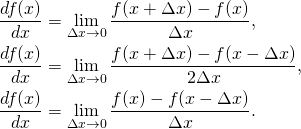However, when working with finite differences the three expressions do differ and are known as the forward, centered, and backward difference, respectively.

If we go through the same steps as for the centered difference above also for the forward and backward differences, we arrive at matrices that are multiplied by a factor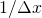instead of. Moreover, the two matrices also have the -1 and 1 off-diagonal entries moved onto the diagonal, respectively. This leads to a different type of boundary conditions once the matrix is truncated. However, here we will not be interested in the finite difference expression for the first derivative directly. Rather, the forward and backward differences are of interest to us since they allow us to arrive at a simple expression for the second derivative.

### Second derivative

The second derivative of a function is given by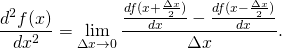When discretized, the first term in this expression can either be understood as the centered difference at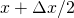, or as the forward difference at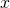. Similarly the second term can be understood as either the centered difference at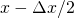, or as the backward difference at.

It is helpful to consider the first and second term to be the forward and backward differences, respectively, since this allows us to stay on the lattice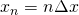. Plugging in the forward and backward differences and removing the limit, we getRewriting this on matrix form we then arrive at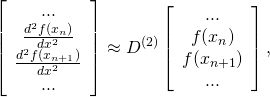where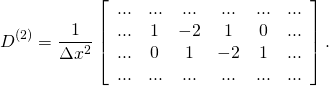Here the -2 entries fall on the diagonal. Truncating this matrix implies the same boundary conditionsas for the centered difference.

## Discretizing the Schrödinger equation

We are now ready to discretize the Hamiltonian of the one dimensional Schrödinger equation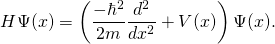Noting that when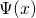is defined on a discrete lattice, the potential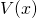becomes a diagonal matrixwith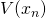along the diagonal. Using this together with the discretized approximation of the second derivative derived above, the discretized Hamiltonian becomes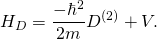## Second quantized formulation

While the equation above is a matrix equation, TBTK is intended for Hamiltonians on second quantization form, which for single particle physics meansHowever, as long as we are concerned with single particle physics, this is really nothing else than a different notation for a matrix with matrix elements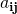. That is,andcan be understood as generalized row and column indices, respectively.

To specify a model corresponding to the finite difference equation above in TBTK, all we need to do now is to think of HoppingAmplitude(value, i, j) as another notation for MatrixElement(value, row, column). We can, therefore, read of the matrix elements of our operators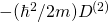anddirectly and feed these to our model. For the diagonal terms, this means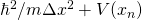, while for the first off-diagonals we find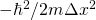. Separating the two diagonal terms, we can implement the model specification in TBTK as follows.

double t = hbar*hbar/(2*m*dx*dx);

Model model;
for(int x = 0; x < SIZE_X; x++){
model << HoppingAmplitude(2*t, {x}, {x});
if(x + 1 < SIZE_X){
model << HoppingAmplitude(
-t,
{x + 1},
{x}
) + HC;
}

model << V[x];
}
model.construct();


Here the variables hbar, m, dx, and SIZE_X, as well as the array V containing the potential is assumed to already have been defined. Also note that only the HoppingAmplitude corresponding to the lower off-diagonal terms is written out explicitly, while the upper off-diagonal terms are included because of “+ HC” at the end of line 12.

## Extention to multiple dimensions

The construction of a multi-dimensional finite difference equation is similar to the procedure described above. However, the function that is being differentiated now depends on several variables that together define a multi-dimensional discrete lattice after discretization. A traditional matrix formulation requires these lattice points to be labeled by a linear index to know which lattice point corresponds to which position in the vectorization of the function, and there are several ways of doing so. The exact form of the derivative operators will, therefore, depend on the convention chosen.

This is a situation in which TBTK shows significant strength since it provides this mapping automatically and allows for the specification of the model using the more natural physical indices. Taking two dimensions as an example, where the physical index is {x, y}, it is simple to extend the one-dimensional case above as follows.

double t_x = hbar*hbar/(2*m*dx*dx);
double t_y = hbar*hbar/(2*m*dy*dy);

Model model;
for(int x = 0; x < SIZE_X; x++){
for(int y = 0; y < SIZE_Y; y++){
//x-direction.
model << HoppingAmplitude(
2*t_x,
{x, y},
{x, y}
);
if(x + 1 < SIZE_X){
model << HoppingAmplitude(
-t_x,
{x + 1, y},
{x,     y}
) + HC;
}

//y-direction.
model << HoppingAmplitude(
2*t_y,
{x, y},
{x, y}
);
if(y + 1 < SIZE_Y){
model << HoppingAmplitude(
-t_y,
{x, y + 1},
{x, y}
) + HC;
}

model << V[x][y];
}
}
model.construct();


The code is almost identical to the code for the one-dimensional case, and for clarity, we have here allowed for different step lengths along the x and y directions.

## Final words

Finite differences is the most straight forward way to convert a differential equation into a form suitable for numerical calculations. We have only scratched on the surface here by for example only focusing on the boundary conditions. We have also left out higher-order approximations and non-uniform grids. However, we have covered enough material to provide a full understanding of the essential nature of finite differences, as well as demonstrated how TBTK makes it particularly simple to work with these also in higher dimensions.

Since it is so simple to set up finite difference schemes in general using TBTK, it can be of interest beyond the application to quantum mechanics. If you have an interested in finite difference schemes more generally and want to know more about how TBTK can be used to simplify such calculations, then see for example how to set up complex geometries and how to extract the matrix formulation of the problem from the model.

In this post, we have only provided two code fragments to demonstrate the principle. For examples of the application of finite differences to solve concrete quantum mechanical problems, see for example:

Using TBTK to calculate the density of states (DOS) of a 1D, 2D, and 3D square lattice

1.Frederick Moxley says: